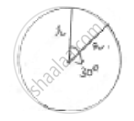Share

# The Minute Hand of a Clock is √21 𝑐𝑚 Long. Find Area Described by the Minute Hand on the Face of Clock Between 7 Am and 7:05 Am - CBSE Class 10 - Mathematics

ConceptPerimeter and Area of a Circle

#### Question

The minute hand of a clock is √21 𝑐𝑚 long. Find area described by the minute hand on the face of clock between 7 am and 7:05 am

#### SolutionRadius of minute hand (r) = √21 𝑐𝑚

For 1hr = 60 min, minute hand completes one revolution = 360°

60 min = 360°

1 min = 6°

From 7 am to 7:05 am it is 5 min angle subtended = 5 × 6° = 30° = 𝜃

Area described =theta/360^@ × pir^2

=30/360×22/7× 21

=22/4= 5.5 cm2

Is there an error in this question or solution?

#### Video TutorialsVIEW ALL 

Solution The Minute Hand of a Clock is √21 𝑐𝑚 Long. Find Area Described by the Minute Hand on the Face of Clock Between 7 Am and 7:05 Am Concept: Perimeter and Area of a Circle.
S